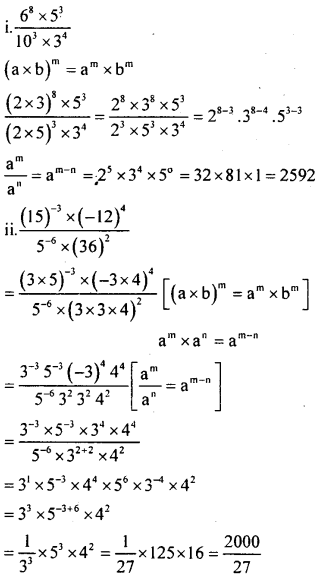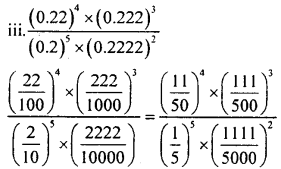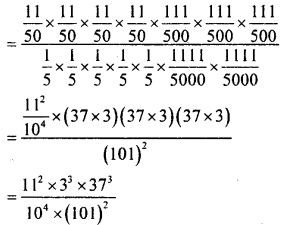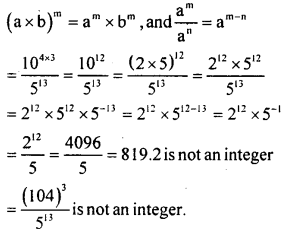# KSEEB Solutions for Class 8 Maths Chapter 10 Exponents Ex 10.5

Students can Download Maths Chapter 10 Exponents Ex 10.5 Questions and Answers, Notes Pdf, KSEEB Solutions for Class 8 Maths helps you to revise the complete Karnataka State Board Syllabus and score more marks in your examinations.

## Karnataka Board Class 8 Maths Chapter 10 Exponents Ex 10.5

Question 1.
Simply
i.  68x53
ii. $$\frac{(15)^{-3} \times(-12)^{4}}{5^{-6} \times(36)^{2}}$$
iii. $$\frac{(0.22)^{4} \times(0.222)^{3}}{(0.2)^{5} \times(0.2222)^{2}}$$Question 2.
Is ∴ $$\frac{\left(10^{4}\right)^{3}}{5^{13}}$$ an integer? Justify your answer.